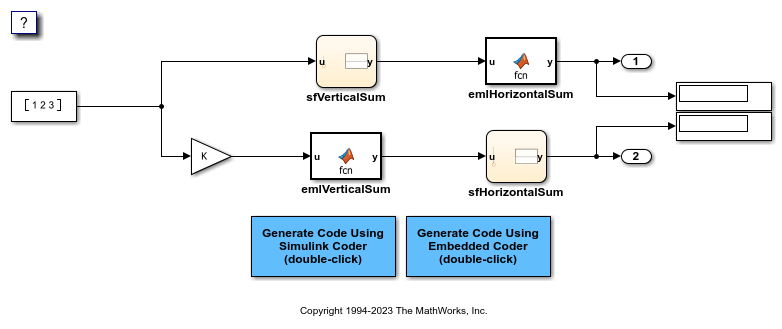# Share Data Between Code Generated from Simulink, Stateflow, and MATLAB

Stateflow and MATLAB Coder can fully define their data definitions, or they can inherit them from Simulink. Data definition capabilities include:

• Inheriting input/output data types and sizes from Simulink.

• Parameterized data types and sizes. That is, data type and size may be specified as a function of another data's type and size, e.g., `type(y)=type(u)` and `size(y)=size(u)`.

• Inferred output size and type from Simulink via signal attribute back propagation.

• Parameter scoped data, which allows referencing Simulink parameters in Stateflow and MATLAB.

### Open Example Model

Open the example model `rtwdemo_dynamicio`.

```open_system('rtwdemo_dynamicio') ```### Instructions

1. Compile the model (on the Simulation tab, under Prepare, click Update Model) and note the displayed signal types and sizes.

2. Change the data type and/or size of the Constant block and recompile the model. Note that the attributes of the signals automatically adapt to the Constant block specification.

3. Generate and inspect code using the blue buttons in the model. Note that `K` is shared by the Gain and sfVerticalSum block.

### Notes

• The data type and size of Stateflow and MATLAB data is inherited from Simulink.

• The Gain block and the Stateflow chart sfVerticalSum share the Simulink parameter `K`, which is defined in the MATLAB workspace as a Simulink.Parameter with `Model default` storage class (i.e., `rtP.K` in the generated code).